GeometrizeTwitterBot  1.0 Python Twitter bot for geometrizing images into geometric primitives
dependency_locator Namespace Reference

Module with functionality for locating the Geometrize executable and other dependencies. More...

Functions

def _get_geometrize_absolute_path ()
Gets an absolute path to where we expect to find the Geometrize executable. More...

def get_geometrize_executable_path ()
Gets the absolute path to where we expect to find the Geometrize executable. More...

def geometrize_executable_exists ()
Checks if the Geometrize executable exists. More...

def get_geometrize_image_folder_absolute_path ()
Returns the absolute path the bot image data folder. More...

def get_geometrize_image_file_absolute_path (filename)
Composes an absolute path for an image file in the image data folder. More...

def get_geometrize_script_folder_absolute_path ()
Gets an absolute path to the bot scripts folder. More...

Reads a Chaiscript script file at the given location, returning the text content of the file. More...

Reads a Chaiscript script file out of the Twitter bot scripts folder, returning the text content of the file. More...

Detailed Description

Module with functionality for locating the Geometrize executable and other dependencies.

◆ _get_geometrize_absolute_path()

 def dependency_locator._get_geometrize_absolute_path ( )
private

Gets an absolute path to where we expect to find the Geometrize executable.

10  baseDir = os.path.normpath(os.path.join(os.path.dirname(__file__), "../geometrize/")).replace('\\', '/')
11
12  possibleExecutables = [name for name in os.listdir(baseDir) if re.match(r'[G|g]eometrize.*', name)]
13
14  if len(possibleExecutables) > 1:
15  print("Warning, found multiple Geometrize executables: " + str(possibleExecutables))
16
17  if not possibleExecutables:
18  print("Failed to find a path to the Geometrize executable")
19  if platform == "linux" or platform == "linux2" or platform == "darwin" or platform == "win32":
20  return baseDir + "/" + possibleExecutables
21  else:
22  print("Warning, the Geometrize bot might not work on this OS")
23
24  return baseDir + "/" + possibleExecutables
25
26
Here is the caller graph for this function: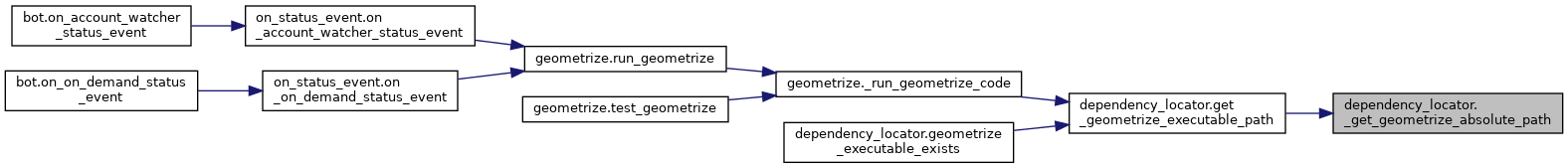◆ geometrize_executable_exists()

 def dependency_locator.geometrize_executable_exists ( )

Checks if the Geometrize executable exists.

Returns true if the Geometrize executable exists at the expected location, else false.

34  return os.path.exists(get_geometrize_executable_path())
35
Here is the call graph for this function: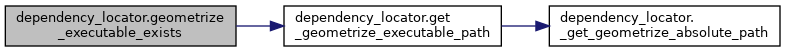◆ get_geometrize_executable_path()

 def dependency_locator.get_geometrize_executable_path ( )

Gets the absolute path to where we expect to find the Geometrize executable.

Here is the call graph for this function: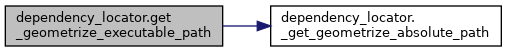Here is the caller graph for this function: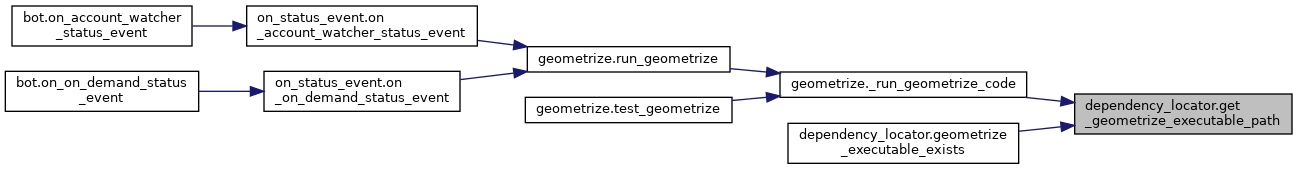◆ get_geometrize_image_file_absolute_path()

 def dependency_locator.get_geometrize_image_file_absolute_path ( filename )

Composes an absolute path for an image file in the image data folder.

43  filepath = os.path.normpath(os.path.join(get_geometrize_image_folder_absolute_path(), filename)).replace('\\', '/')
44  return filepath
45
Here is the call graph for this function: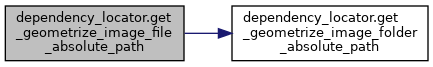Here is the caller graph for this function: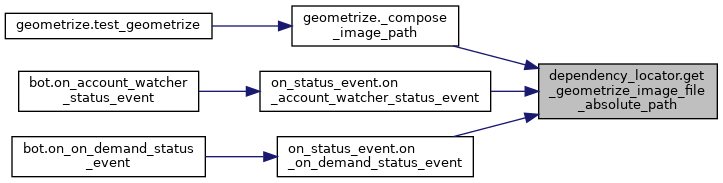◆ get_geometrize_image_folder_absolute_path()

 def dependency_locator.get_geometrize_image_folder_absolute_path ( )

Returns the absolute path the bot image data folder.

38  baseDir = os.path.dirname(__file__)
39  return os.path.normpath(os.path.join(baseDir, "../image_data/")).replace('\\', '/')
40
Here is the caller graph for this function: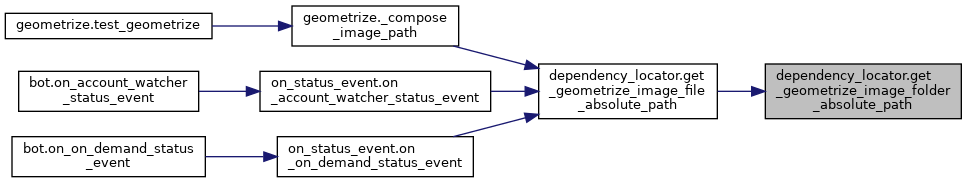◆ get_geometrize_script_folder_absolute_path()

 def dependency_locator.get_geometrize_script_folder_absolute_path ( )

Gets an absolute path to the bot scripts folder.

48  baseDir = os.path.dirname(__file__)
49  return os.path.normpath(os.path.join(baseDir, "../script/")).replace('\\', '/')
50
Here is the caller graph for this function: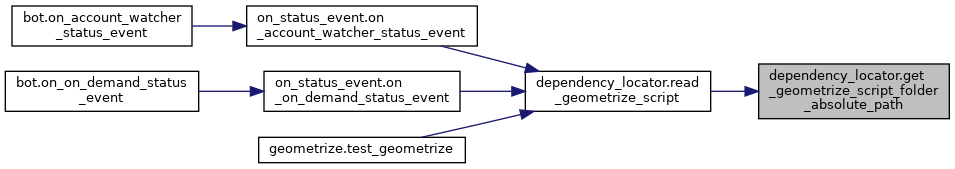Reads a Chaiscript script file out of the Twitter bot scripts folder, returning the text content of the file.

:return An empty string if we failed to read the script.

64  filepath = os.path.normpath(os.path.join(get_geometrize_script_folder_absolute_path(), filename)).replace('\\', '/')
Here is the call graph for this function: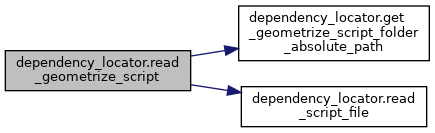Here is the caller graph for this function: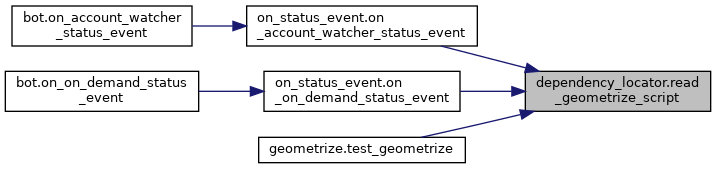Reads a Chaiscript script file at the given location, returning the text content of the file.

:return An empty string if we failed to read the script.

54  with open(filepath, 'r') as content_file:
56  return content
57
58  print("Failed to read Geometrize script at: " + filepath)
59  return ""
60
Here is the caller graph for this function: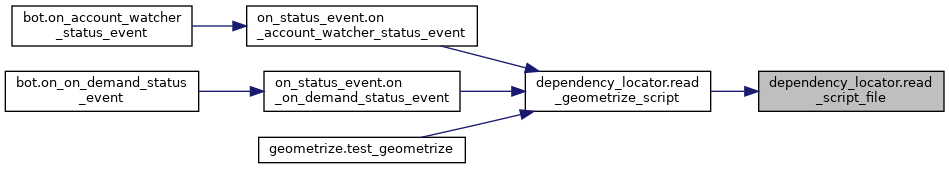dependency_locator.get_geometrize_executable_path
def get_geometrize_executable_path()
Gets the absolute path to where we expect to find the Geometrize executable.
Definition: dependency_locator.py:28
Reads a Chaiscript script file at the given location, returning the text content of the file.
Definition: dependency_locator.py:53
dependency_locator.get_geometrize_image_file_absolute_path
def get_geometrize_image_file_absolute_path(filename)
Composes an absolute path for an image file in the image data folder.
Definition: dependency_locator.py:42
dependency_locator.geometrize_executable_exists
def geometrize_executable_exists()
Checks if the Geometrize executable exists.
Definition: dependency_locator.py:33
dependency_locator._get_geometrize_absolute_path
def _get_geometrize_absolute_path()
Gets an absolute path to where we expect to find the Geometrize executable.
Definition: dependency_locator.py:9